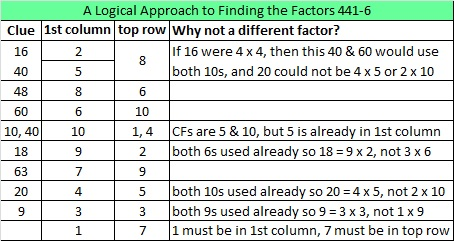# 267 and Level 6

• 267 is a composite number.
• Prime factorization: 267 = 3 x 89
• The exponents in the prime factorization are 1 and 1. Adding one to each and multiplying we get (1 + 1)(1 + 1) = 2 x 2 = 4. Therefore 267 has 4 factors.
• Factors of 267: 1, 3, 89, 267
• Factor pairs: 267 = 1 x 267 or 3 x 89
• 267 has no square factors that allow its square root to be simplified. √267 ≈ 16.3401Can you solve this level 6 puzzle? Possible steps to logically arrive at the solution are given at the end of the post.Print the puzzles or type the factors on this excel file: 10 Factors 2014-10-13This site uses Akismet to reduce spam. Learn how your comment data is processed.